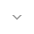belongs to Maven artifact com.android.support:recyclerview-v7:28.0.0-alpha1

# DiffUtil

``` public class DiffUtil ```
``` extends Object ``` ``` ```

 java.lang.Object ↳ android.support.v7.util.DiffUtil

DiffUtil is a utility class that can calculate the difference between two lists and output a list of update operations that converts the first list into the second one.

It can be used to calculate updates for a RecyclerView Adapter. See `ListAdapter` and `AsyncListDiffer` which can compute diffs using DiffUtil on a background thread.

DiffUtil uses Eugene W. Myers's difference algorithm to calculate the minimal number of updates to convert one list into another. Myers's algorithm does not handle items that are moved so DiffUtil runs a second pass on the result to detect items that were moved.

If the lists are large, this operation may take significant time so you are advised to run this on a background thread, get the `DiffUtil.DiffResult` then apply it on the RecyclerView on the main thread.

This algorithm is optimized for space and uses O(N) space to find the minimal number of addition and removal operations between the two lists. It has O(N + D^2) expected time performance where D is the length of the edit script.

If move detection is enabled, it takes an additional O(N^2) time where N is the total number of added and removed items. If your lists are already sorted by the same constraint (e.g. a created timestamp for a list of posts), you can disable move detection to improve performance.

The actual runtime of the algorithm significantly depends on the number of changes in the list and the cost of your comparison methods. Below are some average run times for reference: (The test list is composed of random UUID Strings and the tests are run on Nexus 5X with M)

• 100 items and 10 modifications: avg: 0.39 ms, median: 0.35 ms
• 100 items and 100 modifications: 3.82 ms, median: 3.75 ms
• 100 items and 100 modifications without moves: 2.09 ms, median: 2.06 ms
• 1000 items and 50 modifications: avg: 4.67 ms, median: 4.59 ms
• 1000 items and 50 modifications without moves: avg: 3.59 ms, median: 3.50 ms
• 1000 items and 200 modifications: 27.07 ms, median: 26.92 ms
• 1000 items and 200 modifications without moves: 13.54 ms, median: 13.36 ms

Due to implementation constraints, the max size of the list can be 2^26.

• `AsyncListDiffer`

## Summary

### Nested classes

``` class``` `DiffUtil.Callback`

A Callback class used by DiffUtil while calculating the diff between two lists.

``` class``` `DiffUtil.DiffResult`

This class holds the information about the result of a `calculateDiff(Callback, boolean)` call.

``` class``` `DiffUtil.ItemCallback<T>`

Callback for calculating the diff between two non-null items in a list.

### Public methods

``` static DiffUtil.DiffResult``` ``` calculateDiff(DiffUtil.Callback cb) ```

Calculates the list of update operations that can covert one list into the other one.

``` static DiffUtil.DiffResult``` ``` calculateDiff(DiffUtil.Callback cb, boolean detectMoves) ```

Calculates the list of update operations that can covert one list into the other one.

### Inherited methodsFrom class ``` java.lang.Object ```

## Public methods

### calculateDiff

`DiffUtil.DiffResult calculateDiff (DiffUtil.Callback cb)`

Calculates the list of update operations that can covert one list into the other one.

Parameters
`cb` `DiffUtil.Callback`: The callback that acts as a gateway to the backing list data

Returns
`DiffUtil.DiffResult` A DiffResult that contains the information about the edit sequence to convert the old list into the new list.

### calculateDiff

```DiffUtil.DiffResult calculateDiff (DiffUtil.Callback cb,
boolean detectMoves)```

Calculates the list of update operations that can covert one list into the other one.

If your old and new lists are sorted by the same constraint and items never move (swap positions), you can disable move detection which takes `O(N^2)` time where N is the number of added, moved, removed items.

Parameters
`cb` `DiffUtil.Callback`: The callback that acts as a gateway to the backing list data

`detectMoves` `boolean`: True if DiffUtil should try to detect moved items, false otherwise.

Returns
`DiffUtil.DiffResult` A DiffResult that contains the information about the edit sequence to convert the old list into the new list.

[]
[]